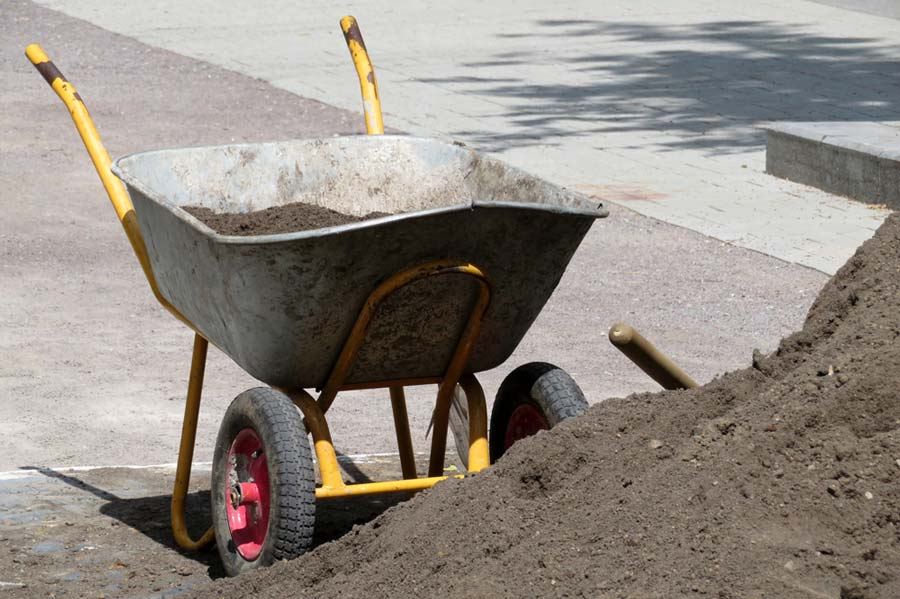# Cubic Yards Calculator

ft in
ft in
ft in
cm
cm
cm
Use this cubic yards calculator to calculate the amount of materials you might need and how much your materials might cost. Materials commonly referenced in cubic yards include concrete, sand, mulch, topsoil and compost.

Disclaimer: Whilst every effort has been made in building this calculator, we are not to be held liable for any damages or monetary losses arising out of or in connection with the use of it. This tool is here purely as a service to you, please use it at your own risk. Full disclaimer. Do not use calculations for anything where loss of life, money, property, etc could result from inaccurate calculations.

## How to calculate cubic yards

The cubic yard is a unit of volume commonly referenced when calculating the amount of materials required for a gardening, landscaping or building project. In order to calculate how many cubic yards of materials you require for your project, try the following:

1. Measure the width, length and depth of the space that you wish to calculate materials for. Note: you must ensure you take your measurements in the same unit (feet or inches or cm, etc) each time
2. If your measurements are not in feet, convert them to feet. Should you need a hand with this, give my length converter a try
3. Multiply your length, width and depth figures together to get an answer in cubic feet
4. Divide your cubic feet figure by 27 (the number of cubic feet in a cubic yard) to give you an answer in cubic yards

If you wish to convert between square feet and cubic yards, you can do so with the square feet to cubic yards calculator.

## How many cubic feet are there in a yard?

There are 27 cubic feet in a cubic yard. This means that if you want to convert from cubic feet to cubic yards, you should divide your figure by 27. We look at this in depth in our article, how many cubic feet are in a yard?

## Materials measured in cubic yards

Materials commonly measured in cubic yards include:

• concrete
• cement
• sand
• aggregate
• rock
• asphalt
• gravel
• dirt
• compost
• mulch
• topsoil## How much will my cubic yard of materials weigh?

If you're looking to find out how much your cubic yard of materials might weigh (in tons, kilograms or pounds), you'll need to do a conversion. The reason for this is because the cubic yard is a unit of volume and the ton, kilogram or pound is a unit of weight. To convert between the two, you'll need to know the density of the substance. To give an extreme example, the total weight of a cubic yard of feathers will be far lighter than a cubic yard of sand.

Should you wish to convert cubic yards to tons, I've built a handy cubic yards to tons calculator which contains density estimates for common materials. It also features an example conversion for a cubic yard of topsoil.